Select Page

## A Child is Born Unto Us

A breeze blows through the 44 palms. On the far side of the oasis, we see Pharoah clutching the limp body of his son. Far above a star shines bright, then explodes like a firework on the 4th of July, into 4 equally bright stars and then all cascade downward. They explode again into 10 twinkling stars each, all slowly trickling down, 44 in total, sparkling and shimmering like a waterfall of light, each gently landing on a different palm.

# כִּי-יֶלֶד יֻלַּד-לָנוּ

“A child is born unto us.” Isaiah 9:5. The word Yeled (ילד), child, has a numerical value of 44, as does the word yalad (ילד) to be born, to bear. The phrase begins and ends with the letters (כו) of numerical value 26, same as the YHVH (יהוה). It is the same as the ordinal value of the child Yeled (ילד). Together they are (44 + 26) = 70, which are the 70 years of the birth pangs of Moshiach.

Now we are in Elim of the 70 palms and 12 springs, the 5th resting place of the Israelites in their 42 journeys from Egypt to Israel.

The initials (כייל) of the phrase “A child is born unto us” also total 70, as in the 70 years of King David at the center of Spherical Time, and the 70 members of Jacob’s family that entered Egypt.

The clear mirror appears as a silvery pool in the night. In the rippling reflection of the moon, we see the words father (אב) and mother (אם) of numerical value 3 and 41, or together 44. It is not the blood moon of the Nile though; it is brilliantly white. Together they are two Alefs (א) and the Mem-Bet (מב) of the 42-Letter Name, the letters in Abraham (אברהם) of numerical value 248.  One letter from each, the first, second, and third respectively, father (אב) and mother (אם) and child (ילד), spells Adam (אדם). The remaining letters are 42.

The child, though, is Moses, referred to as such (ילד) 10 times in the Torah, the last time being in Exodus 2:10, a harbinger of the 10 plagues that would follow and that would end the 210 years in Egypt, 210 years of slavery to physicality. The first of the 10 was when pharaoh ordered the Israelite boys (ילד) to be killed, just as the decree would later fall upon Pharaoh’s own child. Moses was saved by and named after the water of the Nile, where the first plague of blood, dam (דם) of numerical value 44 would begin.

We have already studied the connection of 44, as the regressive Name of Ehyeh (א־אה־אהי־אהיה) of Binah and its connections to Chanukah and the 44 Candles lit over the 8 Days, etc. Now the clear mirror informs us that the word child (ילד) in used exactly 89 times in the Tanakh, as in Chanukah and the 89 days between the Day of Creation and Chanukah on the Cosmic Wheel. Of those 89, 10 were reserved for Moses, leaving 79 or 8.8882, as in (יֶלֶד־יֻלַּד), 88.  You probably should not proceed unless you have read at least Chapter 32, Spherical Time. If you are ready, a whole new world awaits. The Journey begins here if you missed it.

The clear mirror next explains that the milui value of “the child (הילד)” is 543, the complete value of the higher Name Ehyeh Asher Ehyeh (אהיה־אשר־אהיה) and Moses (345) backwards. While the katan value of the Name Ehyeh Asher Ehyeh (אהיה־אשר־אהיה) is 3142, it is found at Exodus 3:14 and the ordinal values of father (אב) and mother (אם) are 3 and 14, which when brought together are both 314 and (3 x 14) = 42.

# כִּי-יֶלֶד יֻלַּד-לָנוּ

With the child (ילד) of numerical value 44, and ordinal values (10 + 16) or 26, we are reminded that in mathematics, the classification of finite simple groups states that every finite simple group is either cyclic, alternation, or in one of 16 families of groups of Lie type, or that it is one of 26 sporadic groups, the outliers, 44 types in total. There are 18 (ידד) total families–Cyclic, Alternating, and 16 Lie types (families) within which we find our E8 Lattice—and then there are 26 outliers, the 26 sporadic groups.

We just learned about the beauty within the symmetry of the 54-digit Monster Group whose digital sum is 216 and that begins with 8080… reflecting the sofit value of the name Abraham (808) and the clear mirror casually mentions that the root (ילד) with the meaning of childbirth is found 54 times within words in Genesis. It also advises us that it is found 19 more times in Genesis with the meaning “child” thus 73 times in total, as in the 73rd Triangular Number that represents the value of the Torah’s first verse. This immediately predates the 10 times yeled (ילד) is associated with Moses. Considering that the value 19 is the back of the Name YHVH (יוד־הא־ואו־הא) associated with Adam/Man (אדם) and Chava/Eve (חוה) and unified with the 44 of Chanukah to give (26 + 19 + 44) = 89, we find it interesting that the root (ילד) is used exactly 19 times in the rest of the Torah, besides the 10 times of Moses, “The Child” who became “The Man.”

Before moving forward, the still pool reminds us that Moses was 80 at the time of the Exodus and at the time of the revelation of the Torah at Mt Sinai. Maybe it is referring to the 8080… Monster Group, but it continues explaining that 82 is 64 as in Adam (45) and Eve (19), which we have already covered, and that the sum of the square roots from 1 to 64 is 345.1307.  The 10 times the root (ילד) was connected to Moses through Exodus 2:10 when he received his name, Moshe (345) plus the 54 times that preceded it are 64.

We see the Sword of Moses of 42 Letters glistening in the shifting pool that moves and shines like liquid mercury, and we are once again shown that its numerical value is 6708, which we know relates to the Inner Core of the Essential Cube of Creation and the Cosmic Wheel. Connecting it to the number 45 and everything associated with it, we learn that the square root of 45 is 6.708204 and that the sum of the square roots from 1 to 45 is 204.398.

## The Cuboctahedron

Nonetheless, what the clear mirror and still silvery pool want to teach us is about a core shape within the 248 dimensions of the E8 Lattice Lie symmetry, the 14-sided cuboctahedron.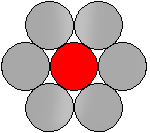In the still silver pool, we see the reflection of the full round moon, 2160 miles wide. As we crane our necks upward, the large bright sphere is surrounded by a ring of 6 identical Moons, all kissing each other with no gaps between them, a distance of 2160 miles from the center of the central sphere to the center of each of the others, and 2160 miles between the central points between each adjacent Moon. Radial or vector equilibrium. We immediately recognize the dynamic of the Cosmic Wheel and the 6 ellipses of the Lunar orbit around the Earth.

We immediately recognize the structure of Zeir Anpin and the One in the center.

They remind us of the 2160 8-orthoplexes in the E8 Lattice. Each of those 8-orthoplex regular 8-polytopes has 16 vertices, 112 edges, and 448 triangle faces for a total of (16 + 112 + 448) = 616, the numerical value of H’Torah (התורה).

In each of the 2160 8-orthoplexes there are 4 triangular faces for each edge, 7 edges for each vertex, and thus 28 triangular faces for each vertex, as in the 28 letters of the Torah’s first verse spread over 7 words, which we know is structured around a set of Triangular Numbers.

The clear mirror wants us to understand that the word H’Torah (התורה), is broken down into (הוה) and (תר) of numerical values 16 and 600, as in 16 and (112 + 448) respectively, and that while the complete value of (הוה) is 32, as in the 32 Paths of the Tree-of-Life, and that the ordinal value of (תר) is 42, as in the 42-Letter Names, the Torah was given to Moses in 2448 HC.

The clear mirror takes a gentler tone as it explains that the mathematics behind the complexity of the Symmetry of the Lie Groups and in particular the E8 Lattice are beyond us and entail understanding an entire language of symbols that represent a range of concepts that only truly make sense in hyperspace. It conveys to us that this is no different than the 22 and 27 Hebrew Letters and their 22 Names.  We see their interaction in the 58 words, letters and verses in the Torah and the 223 rows and columns spread over a 42 x 248 matrix, but individually they are symbols of concepts and operations that can only be truly understood within the dimensionality of hyperspace.

How else to understand why the encasing Pyramid of the 210-cubit Tower of Truth measures precisely 580.0 feet of vertical height from the base of the Subterranean Chamber to the tip of the Pyramid, and that the Primal frequency of the Planck’s Diameter is (27.5 Hz x 21.09143628 x 10-35) = 580.0145…?  Or that the Torah’s total gematria sofit is 5800.0542 or that 580.0 feet is 253.0 cubits of 27.5”, as in the 22nd Triangular Number, the sum of the 22 ordinal values of the Alef-bet?

Or for that matter that the 5 elements of the 248-column Torah quantitatively equal 248,000φ, which is the 3 major Elements (words, letters, and verses) or 58 plus 223?

The clear mirror goes silent, and we can once again focus on the large bright sphere surrounded by the ring of 6 identical Moons, all kissing each other.  The 6 lunar spheres simply form a 6-sided hexagon.

Then we see something brand new.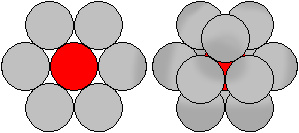Three (3) more identical spheres appear above the hexagon and 3 below forming kissing triangles facing opposite directions. In total 12 spheres packed around the central original One (1), makes 13, echad.  The clear mirror informs us that this is the densest way to pack 13 spheres. No matter the size of the spheres, the counterspace around them is 26%.

All 13 are radially and equilaterally connected, vector equilibrium, forming 12 vertices with equidistant bonds/line segments between them, 24 edges in total. Each bond/edge is the diameter of the sphere, each identical.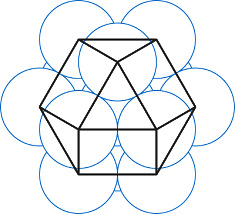Our minds still laser focused of the Cosmic Wheel, the 12 spheres must represent the 12 moons formed by the 6 lunar ellipses and the 24 edges represent both the 24 hours in a day and most specifically the 24 moons (New and Full) formed by the intersecting ellipses.

The clear mirror informs us that the cuboctahedron has 7 planar symmetries, and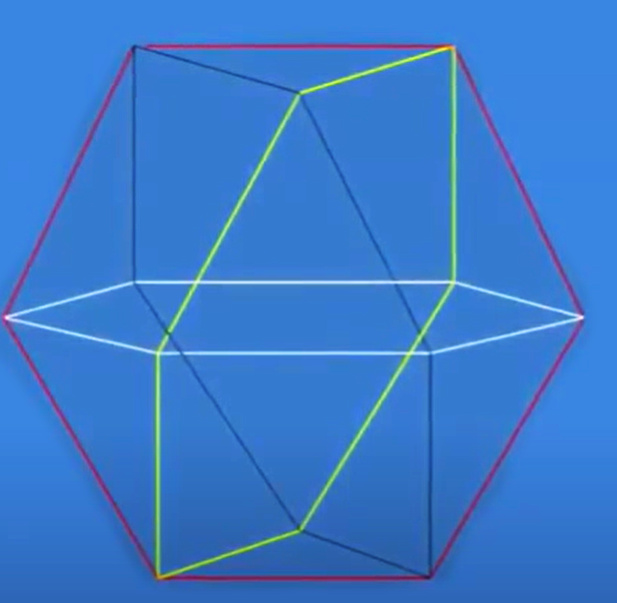suddenly, we know we are correct. The 7 planets built into the Cosmic Wheel must align with the 7 symmetries. The edges highlighted for us, the hexagon horizonal plane is most apparent. Then we see the vertical one and two more angled ones, 4 six-sided hexagons. The cuboctahedron is wholly composed of the 24 edges of the 4 six-sided hexagons.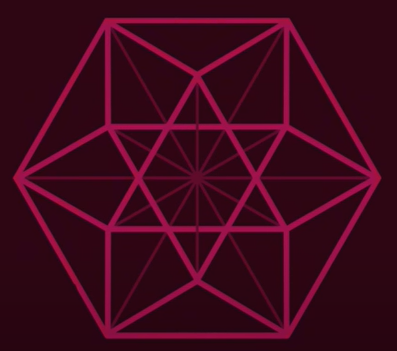And in the center of it all is the 12-vertice Magen David.

With the 12 Moons faded to black, as if in the state of 12 New Moons, the Inner Cosmos made it easier for us to visualize the 4 hexagons. Then it begins a slow spin, first along one axis, then another, 4 axes flashing Magen Davids whenever we see the axis dead on, spinning 360o in multiple directions about the central point of radial symmetry. The axes are 90o or perpendicular to one another. The moons come back momentarily, and we see the 12 Moons carved into 4 seasons or quadrants.

If the 6 ellipses of the Cosmic Wheel are aligned with the hexagon of the cuboctahedron than is the Inner Cosmos telling us the Cosmic Wheel is actually operating/spinning in 4 dimensions rather than a giant dial?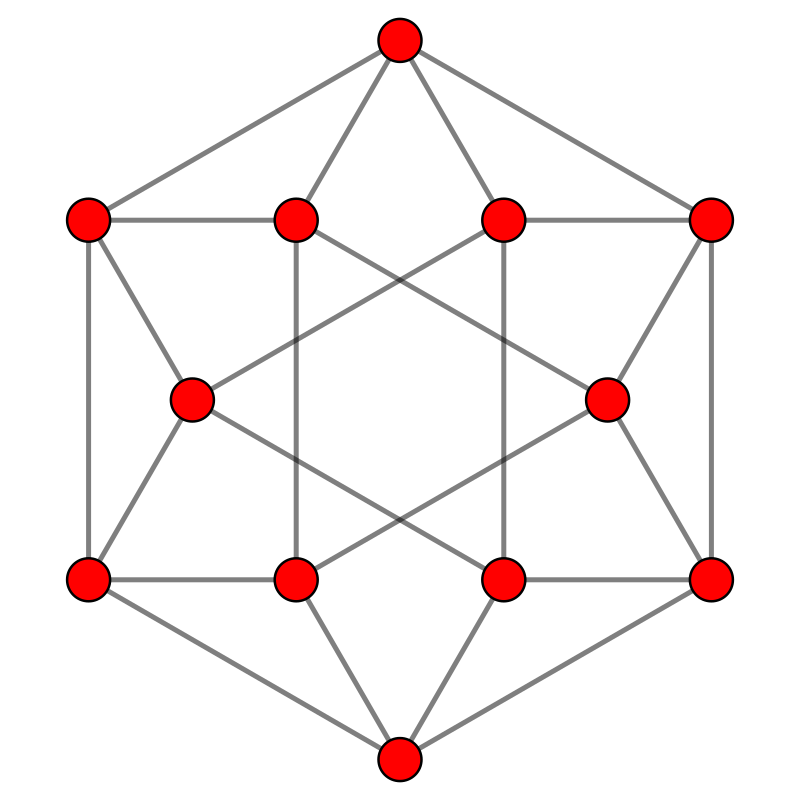Naturally, the 12 spheres of 2160 miles have a total diameter of (12 x 2160) or 25,920 miles, matching the 25,920-year precession of the Earth’s axis and the 25,920 parts of minute in each Hebrew Day.

The clear mirror explains that the cuboctahedron is the root vector polytope of the simplex of the E8 Lattice, and that the 12 vertices in the cuboctahedron can represent the root vectors of the simple Lie group A3.  We hear roots and 12 and blah blah blah and get more excited about discovering that we can form a Magen David out of each of the hexagonal planes just by connecting those 12 vertices.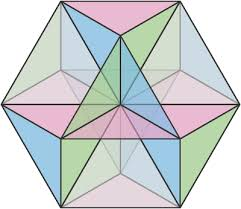As we marvel at this curious shape, the 14-sided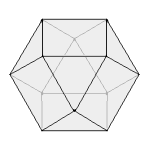Cuboctahedron, comprised of 8 triangular faces and 6 square faces that all have the same edge lengths, the clear mirror shows us that this special object is nothing more than a fully truncated cube, a cube with its 8 corners cut off, hence the 8 triangles, and left over 6 square faces.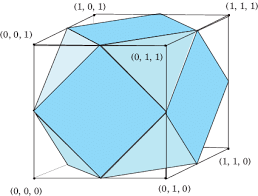In the case of the Essential Cube of Creation this would be the equivalent of eliminating the 8 corners that add up to the 112 Triplets of Creation.

Speaking of the 27 positions of the Essential Cube of Creation, we notice in the still pool that there is now a giant sphere encircling the 13 spheres of the cuboctahedron, fitting perfectly around the various hexagonal belts, kissing all 12 outer spheres. We can see that its diameter is equal to 3 of the inner spheres, the central one plus each of the two that flank it. With the Moon as a guide the diameter is equal to (3 x 2160) = 6480, as in the square root (6.480) of 42, and all the connections we have seen to the structure of the Torah.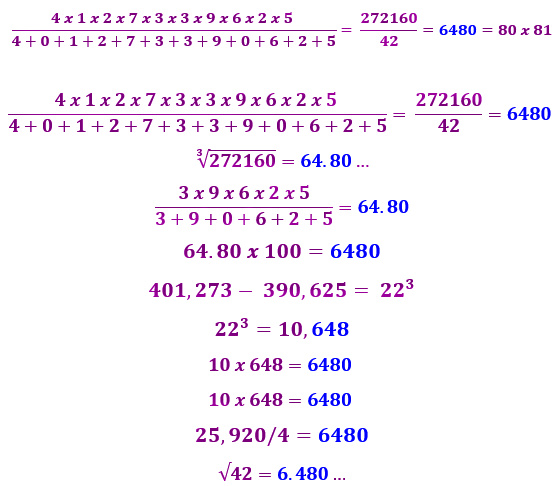With the 4 hexagons dividing the 25,920-distance of the 12 moons into 4 great seasons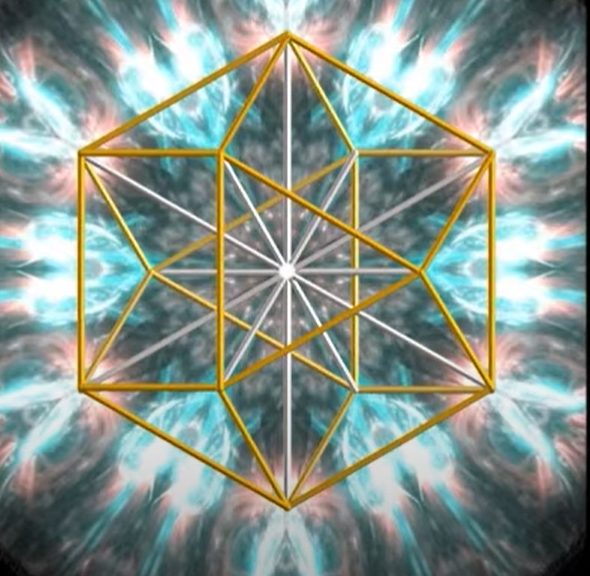of 3 moons each, each set of 3 moons (3 x 2160) is each to the square root of 42, the Source. And each set of 24 hours, One Day, that is 25,920 parts of a minute, making each hexagon ring equal to 6 hours and also the square root of 42.  This explains why the kabbalists divide the Day into 6-hour segments and 6 hours of Ohr HaGanuz were set aside for Moshiach on the 6th Day before Shabbat, while the Israelites fell 6 hours shy in waiting for Moses to return with the Tablets.

If one ring of the Cosmic Wheel applies to the annual cycle of 12 moons, and one ring applies to the Great Cycle of the Precession (12 constellations) and one ring applies to each 24-hour Day, what does the 4th perpendicular ring apply to? We can only surmise that it has to do with Spherical Time.

The giant encapsulating sphere is very apropos, given the spherical nature of time, but what the clear mirror wants to show us is that the ratio of the Major Sphere to any of the inner ones is exactly 27, or 27:1. The ratio of the Major Sphere to all 13 inner ones is 27/13 = 2.07… as in Light, Ohr (אור), of numerical value 207. Together, they represent the ratio of a cube of volume 1 to that of a sphere that circumscribes it, 2.7207. Now that is appropriate from a shape that started out as a cube.

The spheres above us grow in proportion to the edges that are equal to their diameters. And as they grow and shrink in the night sky above us, the still pool of mercury records their various edge lengths, along with assorted stats about the resultant Major Sphere and the enclosed cuboctahedron.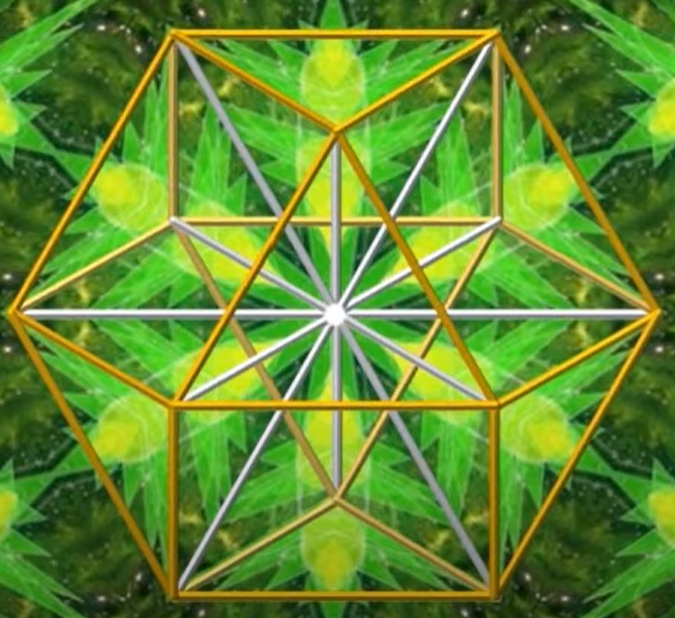The first one highlighted is an odd measurement for a diameter, but most appropriate for the Inner Torah and Spherical Time. The resultant volume of a Major Sphere encircling the 13 spheres of the cuboctahedron with an edge length of the log of 600,000, as in the 600,000 Israelites counted at Mt Sinai, is 2727.276084. The log of 600,000 is 5.778… as in the 5778-year radius of Spherical Time. Within that outer spherical boundary, the 13 inner spheres around the vertices have a total volume of 1313.132929, giving each of the 13 spheres a volume of 101.0102253. Their counterspace difference is 1414.143155.

We are reminded that while the Torah was given to Moses in front of 600,000 men in 2448 HC, it was the year 1313 BCE, and that the exponential curve of the 27 letters of the Alef-bet is (1.313x).

Another, related, yet odd measure for an edge length that the Inner Cosmos highlights is the Speed of Light, 1.86282.  Not only are the 24 edges in the cuboctahedron equal to 44.70… but the volume of the 13 spheres is 44.00020002…, both figures hyper reflective of the child yeled (ילד) and the Spherical Time circumference (363042.4470).The clear mirror points out that besides the 24 edges that connect the 12 vertices there are 24 internal or imaginary edges of the same size that radially connect the outer vertices of each hexagon to the central point. In the case of the Speed of Light, 1.86282, these 48 edges total 89.41…

So, wait!

We know that the difference between the hours in a day of Spiritual Time are 44.70 hours so this kind of makes sense given that the ratio of the Spiritual Time radius over the Physical Time radius is 3760/2018 = 1.86282, which aligns with the 44.70/24 hours.

We then learn that when the Edge length is the speed of light (186,282 mps), the volume of the Major Sphere becomes 91,385,030 miles, off by only 17,000 miles from the Earth’s actual perihelion, its closest point to the Sun in its orbit.

We know that 5778 connects to the Sun, by being its surface temperature, 5778 K, but how did 5.778, as in (3760 + 2018) = 5778 years, turn into 2727.27 and how did the cuboctahedron connect to the 44 and 89 of Chanukah and then everything to the value (44) and complete value (70) of the child (ילד)?  And how does the volume of the 13 spheres equal to 44.000, relating the child to the One fit it?

The verse from Isaiah 9:5 appears again in the still pool, with the 13 glowing spheres and 14th encasing one shinnying bright above us.

## שְׁמוֹ פֶּלֶא יוֹעֵץ, אֵל גִּבּוֹר, אֲבִי-עַד, שַׂר-שָׁלוֹם.

“For a child is born unto us, a son is given unto us; and the government is upon his shoulder; and his name is called Pele-joez-el-gibbor-Abi-ad-sar-shalom;”

Translating the words in his name, we get “His Name is called Wonderful Counselor to Mighty God, the (my) Father of the World to Come, the Prince of Peace.”

The words vanish, though not from our minds, which are still trying to process the message. Nonetheless, the clear mirror advises us that when its edge is of length 6, as is the standard for the illusion of 3-dimensional space in Zeir Anpin, the volume of the Major Sphere, which now fills the still pool with rippling white light, is 6.6612

We know we have seen that number before and the clear mirror obliges us with a reminder that while the sum of the ages of all 26 generations of Adam is exactly 12600, as in the value (126) of each of the planes and of the 6 faces of the Essential Cube of Creation, the sum of their 26 logarithms is precisely 66.6612.

Moreover, with the Earth, representing the 13th or central inner original sphere surrounded by the 12 moons that are associated with the 12 YHVH (יהוה), we note that the Earth orbits the sun at 66,627 mph, only 15 (יה) more than 66612. The clear mirror adds that the sum of the 12 YHVH (יהוה) permutations divided (or filtered) through the 8 vertices of the Essential Cube of Creation is 53328/8 = 6666.

While we know that the Essential Cube of Creation is at the center and core of Creation and thus the universe, we wonder if it is also the original Cube from which the Cuboctahedron was carved.

We have been shown the Major Sphere encasing or bounding the Cuboctahedron with edge length log (600,000), and again with edge length 6 as in the letter Vav (ו) connoting Zeir Anpin, the process of physicality. Now we see it with edge length of the cube root of 6. The Major Sphere becomes 84.82300 or 2 x 4.24115, Moshiach Ben David.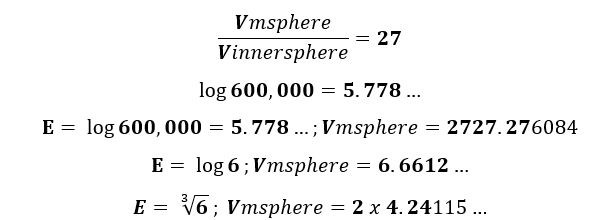The 26 generations clearly refer to the YHVH (יהוה) and the establishment of the 6 dimensions of Zeir Anpin, and so does the Cuboctahedron and its 6 square faces.

What exactly is this shape, this polytope, whose size keeps morphing and keeps relating to the different dimensions of the Inner Torah and Inner Cosmos?

### In the Beginning… the Cuboctahedron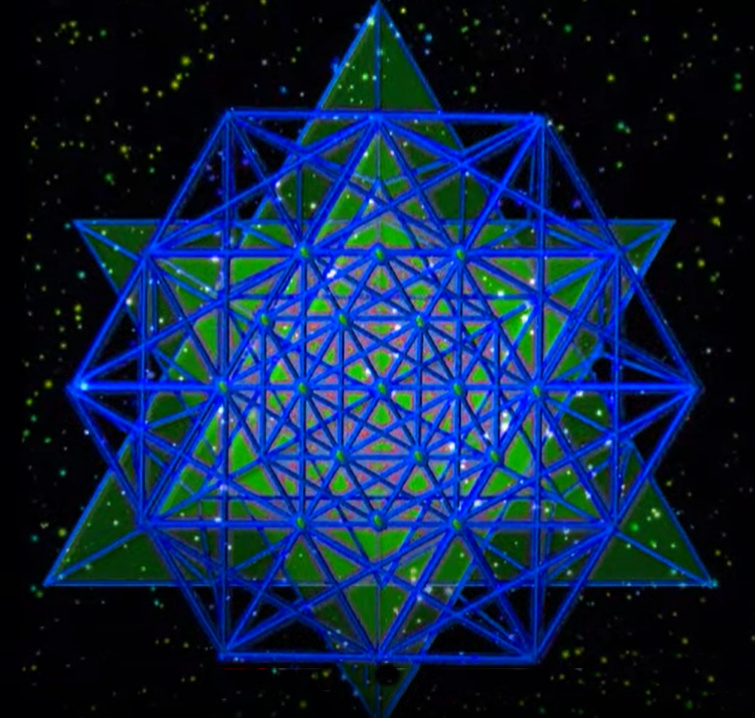We are informed that what we are seeing is the 3-dimensional projection of multiple dimensions of the hyper-cuboctahedron, and that the equation that processes this projection flattens the cuboctahedron with each additional dimension. This means that with each additional dimension the diagonals of the 6 square faces are a different ratio to the standard edge length.  In 76, or more precisely (75.96) dimensions that ratio becomes the square root of Phi (φ).  Since it is scaled up on a curve, it is only equal to the square root of Phi (φ) in that one singular dimension, out of all 248 of the E8 Lattice. We know that the ordinal value of the Torah’s first word, Bereshit (בראשית) is 76, so it makes sense that those 76 dimensions less the 3-dimensional projection, means that the cuboctahedron is scaled up in 73 dimensions, corresponding to the 73rd Triangular Number, 2701, the value of the Torah’s first verse, but what is truly remarkable is that (75.9673) = 2.96, the numerical value of the last word, “The Earth (הארץ), 296 of that same verse.

We can plot the diagonals and the side edges of the cuboctahedron as they change with each Incremental change in edge length and we can do that in each dimension, and they form a stream of curves, one for the edge length and one for the diagonals in each dimension. Yet all those curves intersect in only two points. The first is at the square root of 3 in 2-dimensional space. We have already seen how that comes into play with the Core Equilateral Triangle of sides 346 and height 300 and in particular with the spatial intersection of the diagonal of the side of the Essential Cube and its internal diagonal (Chapter XXXII Part H – The Journey Continues with Creation…).  That is fascinating given the intuitive connection between the cuboctahedron and the truncated Essential Cube of Creation.

The Clear mirror reminds us that a cube whose edge is the √3 is considered the Cube of the 42-Letter Name of small gematria 173, and its volume is naturally (√3)3, synonymous with (3 x the 42-Letter Name).  It has a surface area of (3 x 6) = 18, as in the 18 segments of the bisected Core Essential Triangle of Creation.  Therefore, in scaling up, the volume (√3)3 of the 42-Letter Name Cube is also synonymous with the internal diagonal (√3)3 of the Essential Cube of Creation that is based on 42. The clear mirror further reminds us that they are not just synonymous.  In scaling up, the volume (√3)3 of the 42-Letter Name Cube is the diagonal (√3)3 of the Essential Cube of Creation of 42.

The only other intersection of these two curves is in 3-dimensional space with the square root of Phi (φ).  There is no other intersection in 4-dimensional space or higher.  It is a unique convergence point.  The presence of Phi (φ) as a ratio or primal relationship within the cuboctahedron is extraordinary; it appears to be omnipresent within the Inner Cosmos, as if everything in Zeir Anpin has been filtered through it. What if within the 6 dimensions, the 6 processes of converting the consciousness of Da’at and within it Binah into our holographic projection (Malchut), one dimension consisted entirely of the field of Phi (φ) and another of the field of Pi (π), and everything had to be filtered through them. In 3-dimensional space everything has 3 loci or location points. In 6-dimensional space everything has 6 loci or location points, much like the 6 vertices on the hexagon. For everything that exists, or that we perceive to exist in our world one loci would be situated in the Phi (φ) field and another in the Pi (π) field. That would account for the ubiquitous repetitive and fractal patterns that we find in every level of our physics, mathematics, and 6 senses.

Indeed, what we call nature, and what the Kabbalists call Elohim (אלהים), the 3rd word of the Torah. It was the Baal HaTurim who pointed out that Elohim (אלהים) has the same gematria (86) as H’Teva (הטבע)—which means the natural order. While the ordinal value of Teva, nature (טבע) is 27, representing the 27 positions/letters of the Essential Cube of Creation, the ordinal gematria of H’Teva (הטבע) is 32, or 25, as in the 32 occurrences of the Name (אלהים) in Creation and the 32 Paths of the Tree-of-Life.

With the small gematria of H’Teva (הטבע) being 5927, we have to ask if it relates to the 6 lunar ellipses of 59-days each and the 27 positions/letters of the 6-sided Essential Cube of Creation, both built into the Cosmic Wheel.  It also provokes the question about whether the equation (60005927) = 73 was purposely built into the design of the Torah and Creation.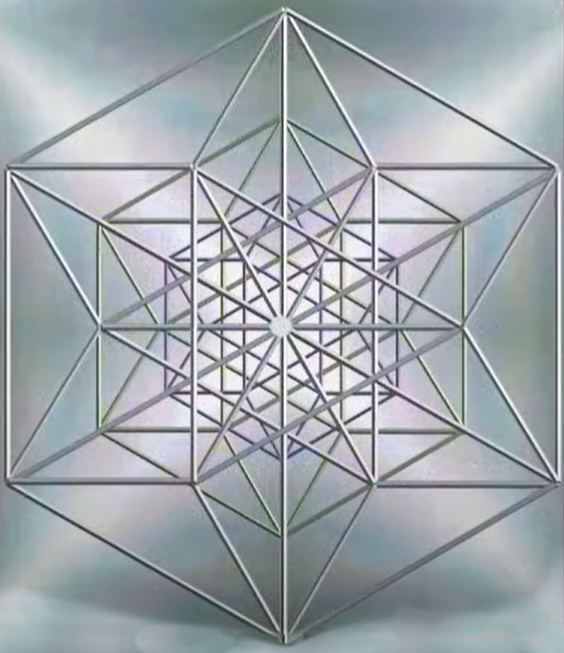It is then that we recall that the cuboctahedron has 7 planar symmetries, as in the 7 words of the Torah’s first verse. Imagining the expanding dimension seems to have expanded our capacity to process or better yet to access Da’at, and as we briefly ponder if  Da’at (knowledge) and data, are related, we notice that the verse’s final 2 words, V’Et H’Ertz (ואת־הארץ), of numerical value 703 represent 26.0% of 2701, meaning the other 5 words represent 74%, as in the most efficient packing of the spheres, in particular the 13 spheres, 26% counterspace (In the Beginning G-d Created) and 74.05%. “In the Beginning G-d Created the Heavens” in the 13 spheres, “and the Earth” in counterspace.

As is known, 703 is the 19th Hexagonal Number and 37th Triangular Number and that 37 is the inner or ordinal value of Chochma (חכמה), Wisdom, the 9th sefira whose outer value is 73 and that (37 x 73) = 2701, the 73rd Triangular Number. The complexity of design that went into producing only the first of the 5845 verses of the Torah never ceases to amaze us.

### Witness Protection

As we further ponder the messages being passed through 73 dimensions of the Beginning and the End, it occurs to us for the first time in this long journey that 2701 backwards is 1072, as in the 107th Triangular Number, 5778.

The still silver pool has much more to show us, but first it adds that 74 represents (עד), the 2 enlarged letters of the 248-word Shema’s first verse that makes up the 45 letters of the 15 Triplets of Creation. Those 2 letters (עד), meaning witness, concealed in gematria atbash (זק) have a numerical value of 107 and are found as the final 2 letters in the 6th line/level of the 42-Letter Name.In case we had any doubts about this connection, the clear mirrors adds together the 2 opposite values (74 + 107) to get 181, the 42nd Prime Number, which is also the sum (181) of the first 42 digits in the set of Prime Numbers. And as we recall, the sum of the digits through 181 is (9 x 42) = 378, which in turn is the sum of the 27 positions in the Essential Cube of Creation.

### The Prince of Peace

It is then though that the phrase reappears in the still metallic pool: his name is called Pele-joez-el-gibbor-Abi-ad-sar-shalom;”

## שְׁמוֹ פֶּלֶא יוֹעֵץ, אֵל גִּבּוֹר, אֲבִי-עַד, שַׂר-שָׁלוֹם.

His Name is called Wonderful Counsellor to Mighty God, the (my) Father of the World to Come, the Prince of Peace.”

The phrase has 9 words and 27 letters, as in the 9 Triplets/planes and 27 positions/letters of the Essential Cube of Creation. Like the numerical value of 48th parsha, Shoftim (שפטים), found at Phi(φ), Devarim, the 5th Book, at 16:18, and like the numerical value of Bereshit Elohim (בראשית־אלהים), “In the Beginning G-d,” the final word in the phrase, Shalom (שלום), Peace, the 9th word in the phrase, has a complete numerical value 999. All 3 words contain (שם), Name of numerical value 900.

Does this hint to the Cube of the 48 Letters of the 12 YHVH (יהוה) that we find in the center of the Essential Cube of Creation and in the structure of the Cosmic Wheel? Does it hint to Spherical Time?

##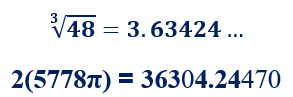The 8th word, Sar (שר), as in Prince of Peace, has the complete numerical value 541, as in Israel.

We have already seen the 7th word, Ad, witness (עד) connected through atbash to 107 and hence the Spherical Time radius 5778. Of course, 541 and 107 together is 648, the square root of 42, but that may be coincidental. Or maybe not, as the 4 initials (אעשש) of My Father Eternal or My Father of the World to Come, Prince of Peace, have a numerical value of 671, as in the 12 letters of the spelled-out Name Adonai (אלף־דלת־נון־יוד), and as in speed of light in mph (671 million mph).

Speaking of atbash, the word Shalom (שלום) is 112 in the atbash cipher, as in the 112 Triplets that form the Cosmic Wheel, along with the 27 letters of the Essential Cube of Creation.

The 6th word, My Father (אבי) has a numerical value of 13 and a complete value of 26, as in the YHVH (יהוה).  It is also comprised of 13 Ahava (Love) plus 13 Echad (Oneness) the same as 1313 BCE, when we received the Torah.

The last 4 words together, words numbers 69, have the numerical value 963, as in (6 x 963) = 5778. The milui of the milui of their collective value is 5424. We are reminded that the spelled-out (milui) value of One, Echad (אחד), is also 963, and that while the initials of the 9 Candles of the 72 Name Matrix are equal to 107, the value 963/9 = 107.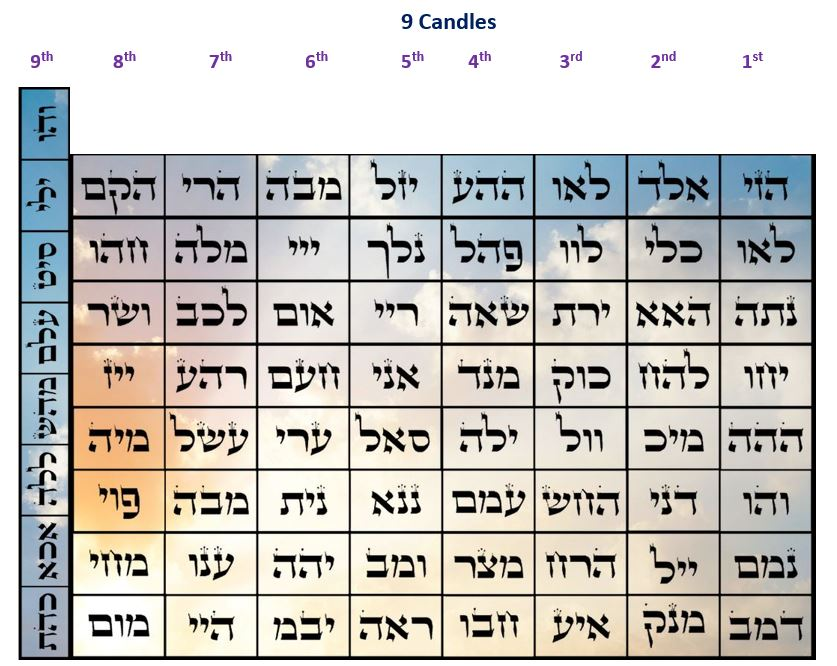### The Spherical Time Diameter

Those same 4 Names, “My Father of the World to Come, Prince of Peace,” stretched across the 12 Moons of the Cosmic Wheel or the 12 surrounding spheres of the cuboctahedron are equivalent to the equation of time since (963 x 12) = 11,556 years, which equals the (2 x 5778) years from the Spherical Time equation: 2(5778π) = 36304.24470, making the time-limit radius in hyperspace equivalent to Echad (אחד), One (1) x 12. One cycle. One pulse of the universe.

To be clear 11,556 years is the diameter of Spherical Time equation and is equal to (12,000444). As we recently learned, 444 is the gematria of the 2nd plague and of “From Generation to Generation (לדר־ודר)” as Rav Ashlag told me, 444/22 (letters) = 20.18181818, reflecting the year 2018 CE (5778 HC) and endlessly reflecting 18. The clear mirror adds that 11,556/444 = 26.270270270…, reflective of the 26 generations through Moses and the Torah, and endlessly reflecting the 27 positions/letters. Moreover, it adds that 11,556/42 (The Source) = 275.142857 or the Prime Frequency 27.5 Hz and 1/7th.

### שְׁמוֹ פֶּלֶא יוֹעֵץ, אֵל גִּבּוֹר, אֲבִי-עַד, שַׂר-שָׁלוֹם.

Given the aforementioned connection to the plague is interesting that the 4th word, God, El (אל) has a complete value of 44, as in the first plague. The Name El (אל) also has the EB-GD cipher value of 42 and an atbash one of 420, as in 420 x 27.5 (plus 1/70th) Hz = 11,556.

It is paired up with the word Mighty Gibor (גבור) to form the Name “Mighty God (אל־גבור)” with an EB-GD value of 314, as in Pi and Shadai (שדי), and Mettatron. With the kolel for their 6 letters, the two-word Name has a numerical value of 248.

The 3rd word, Yoetz (יועץ) has a value of 176, which we know from the magical mirrored matrix connected to the 16 elements and to the longest portion in the Torah, Tehillim and Talmud, but given that, its EB-GD value of 107 is most telling.

The 2nd word Peleh (פלא), Wonderful, is Alef (אלף), 1, backwards as we find at the tip of the 42-Letter Sword of Moses in Exodus Chapter 15, paragraph 42.

The 9-word phrase of the Names of the son, begins with the letters in Shemo (שמו) as it ends with the same ones in Shalom (שלום), except “Peace” includes the Lamed (ל) of complete numerical value 42, and Shemo’s (שמו) numerical value is 2 x 173, the katan value of the 42-Letter Name. The value of Shalom (שלום), 376, is related to the 3760-year radius of Spiritual Time. Moreover, its complete value less the kolel for the 4 letters is 424.

Once again, the verse fades away, and the 14 spheres of the cuboctahedron fill the deep dark sky, casting a glistening sheen on the 70 palms swaying about the oasis. One of those mathematical symbols that connect to higher dimensional hyperspace mathematics are square roots, indeed roots of all denominations. This is why they turn up in so many of the Inner Cosmo’s equations. Each number has a self-reflecting root extending back into the hyperdimensional fields from which it came. We see them as digging down, as we do when we study Torah, but we are actually going upward, expanding our access to consciousness as we do, which is why we can only Understand after we let go of physical boundaries, as opposed to trying to prove a particular observed outcome.

This is why 5778 x .5778, self-referencing, equals 3338, the year the First Holy Temple was destroyed. It can only be understood on a higher dimensional plane. While its message to us may be unclear; it is nonetheless clearly a message.

The clear mirror uses the image of the cuboctahedron and the 14 spheres in the still reflective pool to make a point. First, though, it explains that this dynamic of 13 inner spheres surrounded by 26% counterspace and the 14th or One Major Sphere is not casual, and it has to do with the Divine Summation series 1 – 13 – 91 – 455 – 1820.

# The Blessing of the Kohanim

### Noah’s Ark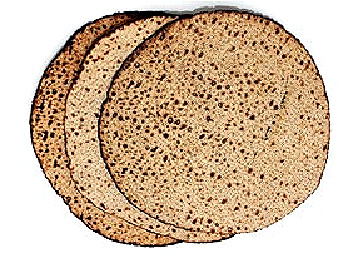The account of the Flood and Noah’s Ark takes place over 3 paragraphs, the 24th, 25th and 26th of the Torah, starting in Chapter 6 of Genesis. Sensing we might need a break from the hyperspace math, the Inner Cosmos shifts gears on us and shows us a different type of hyperspace connection, one seeded with the YHVH (יהוה) that can greatly benefit us while we are still in this world. It is the Blessing of the Kohanim.  Like the 72 Triplets, it is found in 3 consecutive verses in Bamidbar 6:24, 25 and 26. Each of the 3 verses is a level, each of the 3 levels is an expansion.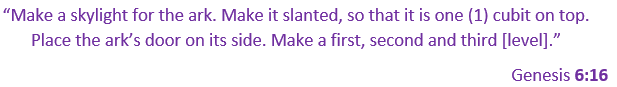Slanted like a truncated pyramid, and like the Altar of the Tabernacle and the Holy Temple, One (1) square cubit on top, One (1) on top that is the Divine Summation series: 1 – 13 – 91 – 455 – 1820.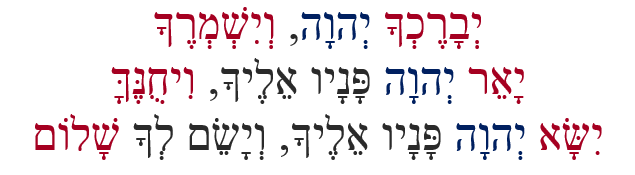“May G‑d bless you and guard you.
‘May G‑d shine His countenance upon you and be gracious to you.
‘May G‑d turn His countenance toward you and grant you peace’.”

The clear mirror is showing us the 3 verses as a symmetric pyramid with the outer (first and last) words highlighted, along with the second word in each verse, the 3 YHVH (יהוה) that form a single Name and powerful meditation unto themselves.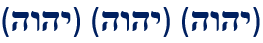In the center on top is the One Name YHVH (יהוה), the One Name that will expand to 1820 YHVH (יהוה) in the Torah, and that is derived from the corresponding 1820 YHVH (יהוה) above in the upper dimensions.The 3 verses begin with 3 Yuds (ייי) of numerical value 30, or 10 (י) cubits per each of the 3 level.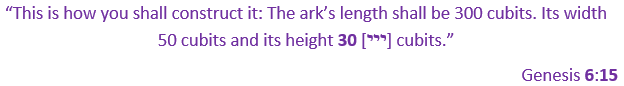Without hesitation the clear mirror tells us that the 6 outermost or encasing words total exactly 1820, matching the 1820 YHVH (יהוה) in the Torah. These are followed by the 3 YHVH (יהוה) that total 78, or 156 in complete gematria, and then the remaining 6 words that total 820, as in the numerical value of the key verse Vayikra 19:18 about loving thy neighbor as thyself. This reminds us of the 1819th prime, 15601 but the clear mirror wants us to concentrate on the 15 words and 60 letters of the blessing.

To draw down the blessings upon us we must keep in mind all 1820 YHVH (יהוה) within the Torah and specifically the 3 in the 3 verses, together. The Cohen must keep them in mind as well.  We do not have to picture all 1820 YHVH (יהוה), just imagine them in a pulsing energetic connected web, activated and connected to the 3 YHVH (יהוה) from the 3 verses and 3 levels deeper into the consciousness of the universe and connect to those receiving the blessing. Imagine the person(s) receiving the blessing surrounded and energized by them.  In a sense you are restoring the connections they already had.

The clear mirror next draws our attention to the initials of the 6 inner words whose complete value is 252 or 6 x 42, as in the Sword of 42 in the center of the Essential Cube of Creation and found in chapter 15 of the Book of Exodus, Shemot, which actually means Names.  The sum of all the initials and finals letters (570 + 428 + 2) in the 15 words of the 3-verse blessing equals 1000 or 103, while all the middle letters sum to 1720. The clear mirror wants us to understand that 1720 is 100 or 102 less than 1820 and that the 42-Letter Sword of Moses of numerical value 6708 is 101 less than 6718, the sofit sum of the 3 verses of the blessing.

The reason the clear mirror wants us to grasp this connection to 10 is that the total gematria of the 3 verses is exactly 2718, as in 2.718, Napier’s constant, also known as Euler’s Number e, the natural limit found throughout and integral to our base 10 mathematics and critical to both mathematics and physics. Euler’s Number e is the unique number whose natural logarithm is equal to One (1).

The blessing of the Cohen is the natural extension of the Blessing from the One.  It is the Blessing of the One.

Moreover, that same vibrational frequency of Oneness and of the 3 verses and 60 letters of the Blessing, 2718, is also found in the 60 Holy Letters of the thrice expanded Names Ehyeh (אהיה) and YHVH (יהוה).

This is very important to the Inner Cosmos, so it reminds us of the Divine summation path from 1 to 13 to 91 to 455 to 1820, the sums of the sums of the sums, meaning these are not just any 1820 YHVH (יהוה), but a specific set found in a sea of YHVH (יהוה) at a convergence of the fields of Yuds(י), Heis(ה), and Vavs(ו).  While the value 455 in the number series is a 12-simplex, and 1820 is a 13-simplex, the clear mirror wants us to know that (1 x 13 x 91 = 1183 and (1183 + 1820) = 3003. The value of the 11 Triplets of Bereshit, the 8 words and 33 letters that equals the 77th Triangular Number, the fount of Mazal, which we know from the Arizal is equivalent to the 3 YHVH (יהוה) from the 3 verses and 3 levels of the Blessing of Oneness/Unity.

1    1    1     1      1     1      1        1        1         1

1    2    3     4      5     6      7        8        9        10

1    3    6    10    15   21    28     36      45       55

1    4   10   20    35   56    84    120    165    220

1    5   15   35    70  126  210   330    495    715

1    6   21   56   126 252  462   792   1287  2002

1    7   28   84   210 462  924  1716  3003  5005

1    8   36  120  330 792 1716 3432  6435 11440

.

.

.

1   13   91  455  1820

The clear mirror further points out that the summation series of the 3rd order is the set of Triangular Numbers, beginning with 1, then 3, as in the 3 verses found in Bamidbar chapter 6, and 10, as in Napier’s constant or Euler’s Number e, which is the sum of all 15 words.

1    3    6    10    15   21    28     36      45       55

It explains that while the cuboctahedron is a fully truncated cube and can be constructed from the vertices of 13 stacked spheres, it can also be constructed solely from 8 equilateral triangles, and that the 6 squares we see in its design are merely the counterspace to them—the 8 triangles occupy all 12 vertices and all 24 edges. Summing up the 24 edges in each of the first 10 Triangular Numbers we get a final length of 5280, or 1 mile, and the surface area for those (8 x 10) corresponding triangles is 27511.89, an allusion to 1000 cubits of 27.5 inches each, with 11.89 close enough to 12” per foot, as the Hebrew word for 1 and 1000 are identical (אלף).

The clear mirror then moves on to tell us that we must say Amen (אמן) of numerical value 91 replying to each line of the Blessing, as 91 is the midpoint of the Divine summation path (1 – 13 – 91 – 455 – 1820), and the midpoint of the Blessing of the Cohen where the central initials (יפא) add up to 91 both vertically and horizontally.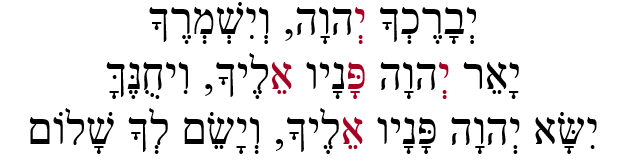With this important realization of our place in the process, the clear mirror explains that 1/(1 + 13 + 91 + 455 + 1820) = 42 = 1/(600,000/70/12/3), reintegrating all 600,000 Israelite souls through the 70 family members and 12 Tribes and 3 Patriarchs through the Names of the Creator to the Oneness and the Singularity of the Aspect of 42, the Divine Source of Creation.  The place of “He and His Name are One.”

The still small voice advises us that we need to use and properly call upon the Blessing more than ever. Through this special Blessing or gift to us that is embedded in the Torah, we can tap into the force and potentiality of unity and unification, a force rarely utilized in 3330 years.

This is so important that the clear mirror further explains that 13 is Echad (אחד) One; 91 is the unification of Adonai (אדני) and the (יהוה); 455 is the value of the 3 Aspects of the higher Names Ehyeh (אהיה); all integrated with the 1820 YHVH (יהוה).  For those that Understand, the 13 represents the 13 Candles of the 112 triplets.

As the clear mirror takes us deeper and higher it explains that together the two sets of expanded Names, Names Ehyeh (אהיה) and YHVH (יהוה) equal (2434 + 708) = 3142, the exact same as the small gematria of “I Am That I Am (אהיה־אשר־אהיה).”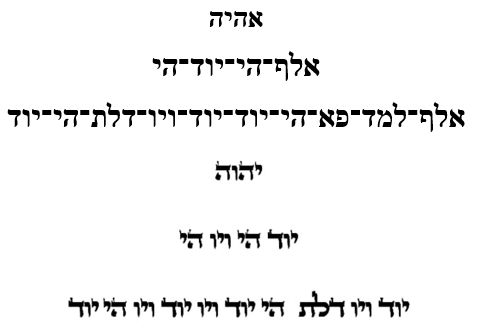When broken down, these include 20 Vavs(ו), 12 Heis(ה), 24 Yuds(י) and 4 Alefs(א), or 60 Holy Letters altogether, out of the (41 + 42) total letters. Collectively, those 60 letters total 424, the value of Moshiach Ben David, the second component of the Spherical Time circumference (36304.24470). What this means, though, is that the rest of the letters in the two expanded 3-level Names have a collective numerical value of (3142 424) = 2718, as in the all-important Napier’s Constant (2.718…) and the Blessing of the Cohen.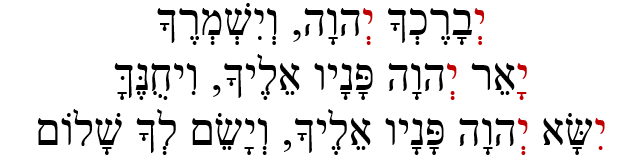And just so we fully understand, the clear mirror equates the 60 letters in the “Blessing” and in the two expanded 3-tier Names and in the 6 initial Yuds (י) with the concealed letter Samech (ס) of numerical value 60 at the center of the 42-Letter Name Matrix that brings its total value to 3760 and One (1).

A subtle breeze blow through the 70 palms and over the 12 springs, whispering, “Here is Wisdom.”

And just so we do not miss the point, it explains that the 1833 Samechs (ס) in the Torah that correspond to the central value of the 42-Letter Name, 1833, is (18331820) = the 1820 YHVH (יהוה) and 13 is Echad, One(1), as in all 13 Candles of the 112 Triplets of Creation and the 13 Yuds (י) in the Blessing of the Cohen.  And as a cherry on top of the sundae, the clear mirror adds that the square root of 336, as in the 336 letters in the 112 Triplets, is 18.330.

The value 1833 is 3 x 611, the numerical value of the Torah, and the 3 Alef (אאא) initials of (אהיה־אשר־אהיה) with the kolel (3) have a spelled-out numerical value of (111 x 3 + 3) = 336, the square of 18.330. Meanwhile, the cuboctahedron of edge length 611 has a spherical diameter of 1833.

If Wisdom can have depth then the less subtle rusting of the palm branches whispering again, “Here is Wisdom” would indicate that this is even deeper Wisdom.

Clearly, the Blessing of the Cohen, the Unification of the two expanded Names, and the powerful Name found at Exodus 3:14 and aligned with the center of the 42-Letter Name Matrix with 21 letters on either side of it, just as in (אהיה־אשר־אהיה) with the Name Ehyeh (אהיה) of numerical value 21 and complete value 42 as wings on either side, all converge on a single point, a singularity.

## I Am That I Am

The highest Name (אהיה־אשר־אהיה) has (21 + 21) = 42 on its wings; 42 as the complete value of each wing (אהיה); and 212, Truth as its’ product; 42 as the ordinal value of the Name (אשר) in its center; 42/2 as the ordinal value of the 21st letter (ש) in its heart, the value of (אהיה), with another 21 as the ordinal value of the 1st and 20th letters (אר), the wings of the heart, as in the 120 stacked cubes of the Tower of Truth (21)2; 210, the height of the Tower and length of the exile that the Name (אהיה־אשר־אהיה) ended, that equals (10 x 21) and equals (5 x 42) as in the value of its final letters (הרה), spelling out “The Mountain (ההר);” and 42 in its entirety, with its small gematria value being 3142.

Running through the center of (אהיה־אשר־אהיה) are the 5 letters (הי־ש־הי) that equal 330, the base of the encasing pyramid and the value of the time coefficient times the Primal Frequency (12 x 27.5).

While the value of the 3 final letters (ההר) is 210, their milui (spelled-out) value is 540, or (210 + 330). The milui value of the 5 letters (הי־ש־הי) is 430, nefesh, representing the full 430 years of exile advised to Abraham at the age of 70 in the year 2018 that were ended by the Name (אהיה־אשר־אהיה). The 4 outer letters of (הי־ש־הי) have a milui value of 70 and form the Name (יהיה) to be used in the time of Moshiach, from “He and His Name are One.”

### The Cosmic Perspective

The Inner Cosmos are showing us that we can engage that singularity, the nexus-point between the 6 dimensions of Zeir Anpin and the hyperspace reality of the Upper Worlds. It is showing us how.

This is not a blessing that we do on or for something or as a connection between us and the Creator; it is a blessing that we convey on others. On Friday nights we convey it on our children. The Cohanim convey it on the congregation. Harnessing the dimensionality in the Names while reciting the Blessing and selflessly praying for others, we can open the portal to the Upper Worlds and tap into that consciousness for ourselves and for those that are being blessed through us.  The Cohen HaGadol did it for all 600,000, reuniting them with the Source, 42.

Our attention is now drawn to the 9 letters utilized (איוה־דלתפמ) in the 3 levels of the two expanded Names Ehyeh (אהיה) and YHVH (יהוה); 5 regular letters and 4 Holy ones that are found in the original two Names, 5 vs 4, like the battle between the kings that drew in Abraham, who prevailed over them all.

Both the standard and the ordinal values of the 4 Holy Letters (איוה) is 22, as in the 22nd Triplet (ייי) of the 72 Triplets (Names) associated with the 3 initials (ייי) of the 3 verses of the Blessing of the Cohen. Their complete value is 44.

The ordinal value of all 9 letters (איוה־דלתפמ) is (22 + 68) = 90, and their standard value is (22 + 554) = 576 = 242, the same as the 3rd word in the Blessing of the Cohen (וישמרך), making their complete value (576 + 90) = 666.

The clear mirror wants us to Understand so this is Wisdom that the 3 initials (ייי) of the Blessing of the Cohen are the 22nd Triplet (ייי) of the 72 Triplets, the 62nd Triplet overall, as in the 62 Yuds (י) in the 620 letters of the 10 Commandments. The full Names of the 3 Yuds (י) or alternatively their complete value is 60; as in 60 seconds and 60 minutes; as in the 60 factors of the number 5040, which is 7! (factorial) and the sum of the Earth and Moon radii; and as in the 60 letters in the “Blessing of One.”

The allusions are obvious to us about time through the Earth’s 24-hour rotation at 60 minutes per hour and 60 seconds per minute and the Earth’s Precession based on its tilt angle of (90o23.4o) = 66.6o and as the Earth revolves around the Sun at 66,600 mph and that everything connects to the precision and perfection of the Cosmic Clock that the Inner Cosmos has been revealing to us piece by piece.

The clear mirror explains that the first 6 cubes of the Tower of Truth, or alternatively the final 6, correspond to the sum of the cubes from 1363, as in the 6 dimensions of Zeir Anpin,. They equal 91 from the Divine Summation series of One, and when the edge of the cuboctahedron is 66.66, its area is 42054.26173… And when the edge of the cuboctahedron is the cube root of 6, its volume is 14.14213… or 102 and the volume of each of the 13 spheres is exactly Pi (π).

The square root of 6 cubits of 27.5” as the edge of the cuboctahedron yields a volume of 720424.8828, as in the 72 Triplets and the 4248 value of the 22 Names of the Alef-bet, etc. And referring to the 72 Triplets, the same edge length also yields 1143.154 as the sum of the 12 diagonals of the 6 square faces, which is the value of the 9th Candle, the first row of the 72 Name Matrix. The volume of the individual 13 spheres are each 160038 or 400.04752, and the volume of the Major encircling Sphere is 4321030, referencing the 432 HZ frequency of Binah and tying in the 210 cubits/frequency of the Tower of Truth.

With the connection of the Name (אהיה־אשר־אהיה) to the Tower of Truth and the sum of the cubes from 13203, the depth of the connection of the 6 combined iterations of the 2 Names Ehyeh (אהיה) and YHVH (יהוה) to 24 is enhanced by the sum of the cubes from 13243 that equal 90,000, as in the ordinal value of their 9 letters that equal 90, and as 3002, the length of Noach’s ark, reminding us that as with hyperspace geometry, the expansion of the Letter and Names by squaring, cubing, and higher powers is what is most important.

The simple effects upon our physical world do not happen unless the complicated mathematics happens first in the higher dimensions.  As complex as the 3-dimensional cuboctahedron is it is just a projection from multiple dimensions above. This is why so many of the edge variations give rise to Torah and Spherical Time equations.

The clear mirror then explains that while 1/(1 + 13 + 91 + 455 + 1820) = 42, the sum (1 + 13 + 91 + 455 + 1820) = 2380, or 10 times the aspect of Rachel (238) in the 10 sefirot (dimensions). Moreover, 1820 is 10 times the aspect of Jacob (182) in the 10 sefirot (dimensions), and the loving union of Rachel and Jacob is (2380 + 1820) = 4200. The Divine Aspect of 42 has always been at the center of it all. It is the singularity.Slanted like a truncated pyramid, and like the Altar of the Tabernacle and the Holy Temple, One (1) square cubit on top, the One (1) on top that is the Divine Summation series: 1 – 13 – 91 – 455 – 1820 is 42.

It has been explained to us by the Arizal that in this hyperdimensional spiritual union, Rachel is the back of Jacob and that they are One, but the clear mirror stresses the sum of the 9 letters, 666, and the further explanation of the Arizal that Rachel comes up to the chest (Tiferet) of Jacob metaphorically, specifically 2/3 (.666) of the way.

Before moving on the clear mirror flashes us Zachariah 13:8 and 9: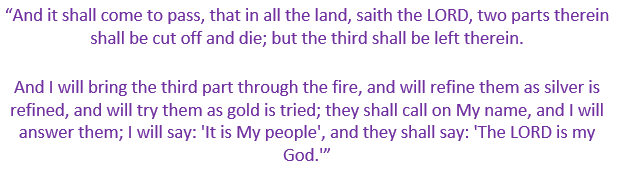And then back to the Blessing of the Cohen connection to the singularity at the center of Spherical Time, where the clear mirror explains that the sum of the 3 levels of the expanded Name, Ehyeh (אהיה) equal 2434 and (24342380) is 54, which turns up in the Inner Cosmos equations as much as 107 does, which makes sense since 54 x 107 = 5778, the Spherical Time radius.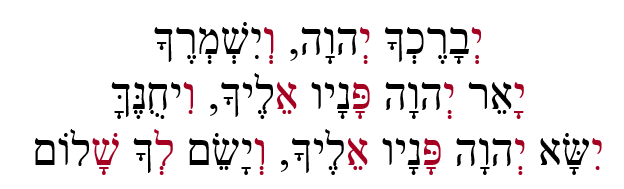The 3 levels corresponding to the 3 verses, as initials break down into (ייו) as 26, as in the value of the YHVH (יהוה) for the first level; (ייפאו) or 107, as in the Spherical Time radius, 5778 years, the 107th Triangular Number for the second level; and (ייפאו) or 107 again plus 330 (לש), which is (12 x 27.5), the Primal Frequency (27.5 Hz) that defines the sacred cubit (27.5”), or alternatively (ייפא) as 101 (Michael), the Protector of Israel, and 336 (ולש), as in the 336 Letters in the 112 Triplets of Creation for the third level.

The still small voice lets us in on another secret: the initials are not just the seeds to the words or verses, but they are the first sounds we hear when the Blessing or Torah is recited; they are the first signals to reach our minds and set the tone for the rest of the word, verse, etc.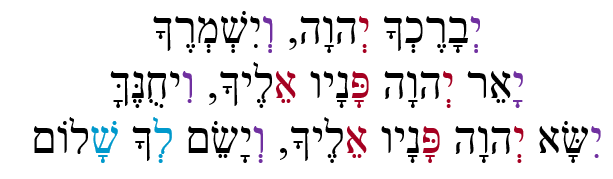The clear mirror shows us the central initials (יפא) that add up to 91 both vertically and horizontally within Blessing of the Cohen, but this time it adds the encapsulating (יו) initials that surround them.  That gives us 13 initials that form 3 sets of 107 or 5778, as in the 3 separate …5778… found in the first 1000 digits in Pi (π), and (לש) of complete numerical value 363, as in H’Moshiach, the 363 Days/Letters in the Cosmic Wheel, and as in the result of the Spherical Time equation: 2(5778π) = 2π(3760 + 2018) = 36304.24470

Returning to the 15 initials of the Blessing, we are shown that while the first 9 initials total 143, the average value of the Triplets in the 9th Candle (first row) of the 72 Triplet Matrix, all 15 collectively total 570, which leads to (3630 + 570) = 4200 from the Spherical Time Equation, 36304.24470, and the Divine Summation Series.

We barely have time to digest that when the clear mirror reminds us that the small gematria of the central initials (יפא) is 181, as in the number 181, which is the 42nd Prime, and that 181 is also the sum of the first 42 digits in the set of Prime Numbers.  Moreover, the sum of the digits through 181 (the 42nd Prime Number) is 378, and 378 is the gematria of the palindromic phrase “He and His Name are One (Echad Usho Echad)”, and while (378 + 42) = 420, there are 181 digits in 111! (factorial) with 111 being the numerical value of Alef(אלפ), One (1).  Indeed, He and His Name are One,” and the Blessing of the Cohen was designed to get us there.

We are left with the impression that it is now or never to latch onto the spiritual time frame within Spherical Time.  The allusions to 5778 were obvious in the 3 sets of the 107, and they are also apparent as 5778 is 3330 years or 66.6 jubilee years after the Exodus and reception of the Torah in 2448 HC, and as Rav Ashlag of blessed memory taught us, 5778 is derived from the same kabbalistic principle of 1/3, 2/3 or .333 and .666 that applied to the metaphysical structure of Rachel and Jacob.  The Torah also made it quite clear to us that the Flood and the Ark to escape it are tied to the Blessing of the Cohanim and the Event Horizon in 5778.

Like the 42-Letter Name gifted to us in the first 42 Letters in the Torah, and the fount of mazal gifted to us in the first 33 letters of the Torah, and the 72 Triplets gifted to us in Shemot, and the Shema in Devarim, the Blessing of the Cohen in Bamidbar is a powerful gift and tool to help us achieve the ultimate goal of connecting our consciousness to the beyond and thus to freedom from the restrictions and illusions of physicality and time. Just because the gifts were left under our tree does not mean they can actually do anything for us. The Inner Cosmos has already unwrapped them, and if you are reading this journey, it has placed them in your hands. It is up to us to engage them with our minds and activate them. Only our minds can connect us to our consciousness. We must consciously decide to open it. Then the gifted tools can go to work for us.

### The 12 Tribes

Like the 28 letters in the Torah’s first verse, the central 3 initials (יפא) of the Blessing of the Cohanim that add up to 91 both vertically and horizontally have an ordinal value of 28, as in the sum of the 6 factors of the number 12.

The clear mirror reminds us that the 54-unit shift in the splitting of the Alef-Bet is equivalent to a 13o shift in a circle, and it now explains that when the edge of the cuboctahedron is the square root of 54, the volume of the 13 spheres is 2701.049942, as in the numerical value of the 7 words of the Torah’s first verse. It further ties this hyperspatial relationship into the Divine Summation Series of One (113914551820) in that 91/7 = 13.

Furthermore, the clear mirror explains that the area of the 8 triangular faces of the cuboctahedron with that same √54 edge is 187.0…, as in the 187 chapters in the 54 portions of the Torah. The value 1870 is not only the sum of the first 4 powers of 7 but relates to the 1820 YHVH (יהוה) in the Torah through the 2 censuses of the 12 tribes.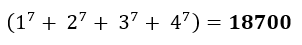The 1820 YHVH (יהוה) in the Torah determine the grouping of the 12 Tribes into 2 groups of 6, similar to the way the number 12 has 6 factors, and the 6 ellipses of the Cosmic Wheel connect to the 12 Moons.  The gematria of the 6 Names of the first group is 1820, and the difference in their net survivors is also 1820. These include Asher (אשר) as in the Name at the center of Ehyeh Asher Ehyeh (אהיה־אשר־אהיה) and Naftali (נפתלי) of numerical value 570, the sum of the 15 initials of the Blessing of the Cohanim.

For whatever reason, the Name Naftali (נפתלי) also has the ordinal value 75, as in the 15 words and 60 letters of the same Blessing. This is also the sum of the 3 paragraphs of Noach and the 3 verses of the Blessing of the Cohanim, (24, 25, and 26) both in the 6th Chapter. Dan, Asher, and Naftali are the 10th, 11th, and 12th Tribes in order of their census recording, 33 collectively, the final 3. The complete value of their 3 Names is 1260, or (30 x 42), as in the 42 times Yehuda (יהודה) of numerical value 30 is mentioned in the Torah, and as in the 126 values of the planes and the faces of the Essential Cube of Creation, and the sum of the ages of all 26 generations of Adam (12600).

Group #1

 Name Name Gematria 1st census 2nd census Difference Reuven רְאוּבֵן 259 46,500 43,730 (2,770) Gad גָּד 7 45,650 40,500 (5,150) Efraim אֶפְרָיִם 331 40,500 32,500 (8,000) Binyamin בִּנְיָמִן 152 35,400 45,600 10,200 Asher אָשֵׁר 501 41,500 53,400 11,900 Naftali נַפְתָּלִי 570 53,400 45,400 (8,000) 1820 262,950 261,130 (1,820)

The gematria of the 6 Names of the second group is 1870 and includes the tribe of Dan of numerical value 54.

Group #2

 Name Name Gematria 1st census 2nd census Difference Shimon שִׁמְעוֹן 466 59,300 22,200 (37,100) Yehuda יְהוּדָה 30 74,600 76,500 1,900 Dan דָּן 54 62,700 64,400 1,700 Yischar יִשָּׂשכָר 830 54,400 64,300 9,900 Zevulun זְבֻלוּן 95 57,400 60,500 3,100 Menaseh מְנַשֶּׁה 395 32,200 52,700 20,500 1870 340,600 340,600 –

The 2 censuses highlight the differences in how many tribe members remained after the 40 years of journeys through the desert. In the second group of tribes, there was zero net differential and the sum of the gematria of their names equals 1870, or 50 more then he first group.  This means that the net differential for all 12 tribes after their sojourn was 1820.

When we add the gematria value for all 12 Tribes, we get (1820 + 1870) = 3690 and then adding 70 for the Israelites who entered Egypt, we get 3760, as in 3760 HC, as in the Spiritual Time radius.

We also note that the total tally for the first set of 6 tribes begin with 26 both times and that the second set of tribes both have the digits 346 as in (2 x 173, the 42-Letter Name).

### Spherical Time With an Edge

The Inner Cosmos then explains to us that the when the √54 is the edge of the cuboctahedron the difference between is volume and surface area is precisely 424.24595, representing 42, the Source, and Mashiach Ben David, the energy consciousness center built into Spherical Time.

The clear mirror wants us to know that when the edge of the cuboctahedron is the √22, as in the 22 Letters, the volume of the sphere is 54.0… and the difference between its volume and surface area is precisely 35.008915… as in the magnitude boundaries of the Planck’s  Diameter and of the pending solar crescendo on the opposite end of the scale, mimicking the 35 years splits in King David’s life (2889 HC +/- 35 years) at the center of the Spherical Time Radius (5778).

We watch as the cuboctahedron above us steadily grows in size and see reflected in the still pool a ratio between its surface area and its volume. As the edge grows longer so does the surface area and the volume. The clear mirror advises us that the ratio grows 24.9049% or 25% with each unit growth but the absolute difference varies considerably.  Oddly, 24.9049 AU is the distance between Jupiter’s moon Thebe and Neptune, the 8th planet. And even odder still, with two years to go before solar maximum Neptune’s atmosphere has just collapsed.  All the planets are tied magnetically to the Sun and as our magnetic fields weaken, the atmospheres become more susceptible to the Sun’s influence.

Less than 1 unit as edge length, the absolute difference naturally shrinks toward 0 the smaller the edge length gets. At 1 unit, the difference between the volume and the surface area is –7.10707, reminding us of the 10 Commandments at Chapter 70 in the Torah and at letter 107,007. The comparison is purely mathematically and exists only in hyperspace as the units represents two different dimensions, square space (a2) and cubic space (v3).

At 4.5 units, that incremental increase in ratio reaches 112.072% but the numerical difference is actually negative until it reaches a minimum of –22.6000 at length 2.7. It hits rock bottom at 2.677, as in the 267-cubit encasing pyramid apothem, and stays negative until the edge length reaches 4, or more precisely 4.015265367 where the numerical difference between the area and volume is about 0.00048.  At 4 the v/a ratio is 4 x 25% = 100% and at 2.67, it is 66.6703%, as in the 66,670 mph speed the Earth orbits the Sun.

Thus 2.67 is a boundary of the cuboctahedron and the clear mirror reminds us that the base measure of the pyramid times 2.6700 = 2018 and that the sum of all the integers from 1 to 267 is 35778, as in the 3 5778’s in Pi though 1000, connecting 5778 HC with 2018 CE, its analogue in the Western Calendar.

### The Path in the Center

The Hebrew word brit (ברית) or Covenant is built into and spelled out sequentially in the central level/line of the 14 Triplets of the 42-Letter name.  This is the central point where the 7 planets and the 6 ellipses and 12 moons orbit about. The position 14 and its corresponding letter Nun (נ) are in the center of the 9 (ט) plane, 3 (ג) axis Essential Cube of Creation based on the number 42.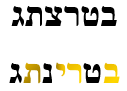The central line/level of the 14 Triplets sitting squarely in on the center of the silver pool, the expanding edge length, with its resulting equations concerning the cuboctahedron and the 14 spheres, grows and shrinks before us, pausing just long enough for the still reflective pool and clear mirror to highlight specific connections, such as the edge length of the cube root of 612 cubits, the value for brit, covenant, like the Creator made with Abraham in 2018 HC, and with the Earth in 1658, exactly 400 or 202 years earlier, as in the base of the Tower of Truth and the 400 silver shekels Abraham paid for the Cave of Machpelah in Ephron’s Field with all its Trees in its circumference at the edge of Mamre.

The value of the cube root of 612 is only .004903 more than the square root of 72, which is twice 4.24, Moshiach Ben David. The still pool shows us that the volume of the cuboctahedron is 29999447.13 inches2 or 543 inches from exactly 30 million, and that the volume of each of its 13 spheres is 6664201.59 inches3 while the shared 48 edges for the 14 triangles and squares measure 11207.04 in total, an allusion to 112, and derech (704) the Path at the enter of the 14 Triplets of the 42-Letter Name. It also matches the ratio 112.07% for the edge length 4.5.

Those 543 inches allude to the winged Name Asher (אשר) in the center of Ehyeh Asher Ehyeh (אהיה־אשר־אהיה) and they are the equivalent of 45.25 feet. The Name Asher (אשר) is found 5 times in the verse (23:17) when Abraham bought the Cave and the round Field (השדה) of numerical value 314, alluding to Pi (π). The root of the field (שדה) found 3 times in that same 20-word verse has a value of 309 and an ordinal value of 30, as in the 30 million inches. And while it too refers to the 3 axis and 9 planes of the Essential Cube of 42, 309 reversed is 903, as in .004903 and as in the 42 Triangular Number.

We must remember that each cubit represents a cycle of the Primal frequency, 27.5 Hz.

Another interesting equation the Inner Cosmos pauses at for us involves the cube root of 2448 cubits, as in the year Moses received the Torah at Mt Sinai (130) and the end of the exile in Egypt. Its resultant cuboctahedron surface area is 1300027.0 inches2 and its volume is 119997788.5 inches3 making the diameter of its encircling Major Sphere 1111.87. The clear mirror is showing us that the cubit and Primal Frequency play an important role in Oneness. There is a purpose and place for everything, and time, nothing more than a broadcasting radius, falls under that maxim.

### Come Sail Away

We have seen how the Torah was given to Moses in front of 600,000 men in 2448 HC, and all the connections that 600,000 and the derivatives of 6 have with the cuboctahedron and Zeir Anpin, etc. including its logarithm being 5.778, or (666 x 50) 3330 years later, with 50 being the height of the Gates of Binah and the Holy Temple and consequently the length of the Jubilee year.

We have seen how 2448 HC is the year 1313 BCE, and that the exponential curve of the 27 letters of the Alef-bet is (1.313x). We have seen how the ratio of the Major Sphere to any of the 13 individual spheres is 27, and now we learn that the ratio of the surface area of the 6 squares to the 8 triangles of the cuboctahedron is 1.732050808 or the square root of 3, which begins with the small gematria of the 42-Letter Name (173), the boom of the sail of the Core Equilateral Triangle, or half its sides. And that its 10th digit ends with 808, Abraham, found in the Monster Symmetry Group.

As a crystalline sailboat floats upon the silver mercury bath with a mast of 300, a boom of 173 and the hypotenuse of the sail of 346, we see the Essential Core Triangle of the Core Equilateral Triangle (Chapter XXXII Part H – The Journey Continues with Creation…)

### Elohim (אלהים)

The value 300 is not arbitrary in the slightest; it the spelled-out value, the value of the 5 Names representing the 5 letters of the Torah’s 3rd word, G-d, Elohim (אלהים).  The fully spelled out Name Elohim (אלהים) has an ordinal value of 120 as in the age of Man and Moses, the child, giving its 13 letters a complete value of 420.

The ordinal value of Nes (נס), miracle, is the same as the spelled-out Name of the letter Lamed (למד), making the ordinal value of Elohim’s (אלהים) first 2 letters Alef(אלפ)-Lamed (למד) equal to (30 + 29) = 59, two lunar months in the Cosmic Wheel, 1 lunar ellipse.

The next spelled-out Name within (אלהים) is the 3rd letter (הי) concealing the Name Yah (יה) of numerical value 15.  It is followed by Yud (יוד) giving the two letters of the Name Yah (יה) a complete value of 35. The final letter Mem (מם) has an ordinal value of 26, as in the Name YHVH (יהוה). Together these 3 letters take on the combined Holy Name Yah-YHVH (יה־יהוה).  These represent the 12th, 13th and 14th letters of the Torah or 42 for the 3 altogether.  The Name Elohim (אלהים) itself represent the 10th, 11th, 12th, 13th, and 14th letters or 60 letters, as in the 60 letters of the Blessing of the Cohanim, and the 60 Holy Letters in the 2 spelled out Names.

What the clear mirror wants us to understand is that Noach’s Ark is 300 cubits long and 50 cubits wide and 30 cubits high, as in the ordinal value of the letter Alef(אלפ).

Egypt, Mizra’im (מצרים) of numerical value 380 represents the enslavement of physicality. The Ark of numerical distance (300 + 50 + 30) = 380 represents Freedom/Salvation through the One (1) cubit skylight. Captured within Egypt, Mizra’im (מצרים) is the Resh-Yud (רי) of numerical value 210, as the height of the encapsulated Tower of Truth, the 210 years of exile within Egypt, and the limits of the Planck’s diameter begging to be released.  It is also the albam gematria of “The Field (השדה),” related to Pi (π).

### The Source of the Primal Frequency

The still small voice, sensing we are ready to take the next step, as we prepare to follow the required 15-step initiation to the Order of Pesach, advises, “Here is Wisdom.”

The Sacred Cubit, the Primal Frequency that permeates the Ether, is 27.5 inches or Hz. This is the Name of Zeir Anpin, YHVH (יהוה) and of Binah, YH (יה) together or (26 + 1.5) = 27.5. This is the Holy Name Yah-YHVH (יה־יהוה).  Yah (יה) is 15, the 15 cubits that covered the Earth and destroyed all breathing flesh upon it. Yah (יה) is the area of the Ark of Salvation (300 x 50) = 1500, the highest word value in the Torah, compared to 3, as in the 3 levels of 10 cubits each, the shortest word value in the Torah.

Could this be why we were elaborately shown the boundary point of the ratio between the surface area and the volume of the cuboctahedron, at edge length 4.015265367. We might as add that we were shown the 40 too as in the 40 days and 40 nights while we are at it, and the 2 periods of 40 days that Moses spent on My Sinai to retrieve the 2 sets of Tablets.  That boundary point is equal to the two measurements in 2 different dimensions being equivalent (4.015265367 x 24.9094) = 99.9997% or 100%.

The clear mirror brings up the 5 Names of the 5 Books that add up to 2480 and the 5 main elements of the Torah Matrix that add up to 401273 or 248,000φ and that are 223 more than the 3 main quantitative Torah elements as we saw with the 6480 equations. It shows us that the boundary point of the central cuboctahedron, 4.015265… is (4.015264.01273) = .00253 more than it, and that 253 is the 22nd Triangular Number, or the sum of the integers from 1 to 22.

When 5 cubits becomes the edge, or the Phi(φ) angle (137.5o) in inches (137.5”), the ratio between the volume and the surface area of the cuboctahedron becomes 3424.420%. The Phi (φ) field always seems to find its way at the heart of all matters and of Time. With the Primal Frequency applied we see it work its magic once again, connecting it to Moshiach Ben David and the Source, 42. We already know that the Phi(φ) angle (137.5o) and Pi (π) fields interact to create the infinite pathways of Spherical Time but for the first time the Inner Cosmos is making a substitution for us in the Spherical Time Equation.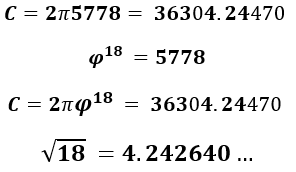### Yah-YHVH (יה־יהוה)

The ratio of 26 to 1.5 is 17.333, the 42-Letter Name, and while it is 10.00 times the square root of 3, it is 15.60128253 more than he square root of 3: as in the 1560 times and 15601 digits that 1808010808 is repeated in the unicorn Prime Number; and as 15601, the 1819th Prime Number; not to mention, Noach’s age at the time of the Flood (601); Joseph (156) and the 12 Tribes; Zion (156), the nexus point of Zeir Anpin; (26 x 60); the Meeting Tent (אהל־מועד) of the Tabernacle; and the 15 words and 60 letters in the Blessing of One.

The clear mirror extends our understanding just a bit further with the equation (15601 + 1819) = 17,420 = (670 x 26) as in the 670 Paragraphs in the Torah, connected to Binah (67), times the YHVH (יהוה). We should not forget either that the 420th YHVH (יהוה) is at the 10 Commandments.

The ratio of 27.5 to 1.5 is 18.333, the center of the 42-Letter Name, the place of the (צר) of ordinal value (2018) in the center of the water mayim (מים) of Egypt, Mizra’im (מצרים), and the square root (18.330) of 336, as in the 336 letters in the 112 Triplets upon which Creation is constructed.

The Holy Components (27.5 + 15) = 42.5 as in the value of the final Triplet (כהת) of the 112 Triplets of Creation, the final Triplet of the 9th Candle of the 72 Name Matrix and thus of the 13 Candles. It is the Name (כהת) that we use on Purim to obliterate the memory and evil of Haman. It is the Triplet that remains lit for 3 hours a day, the last 3, for each of the 8 days of Chanukah, 24 final hours in total, one day, one revolution, as in the 24th paragraph of the Torah, the final paragraph before the Flood, when “All flesh has perverted its way on the earth.”

### Magnetism and the Holy Components

We learned earlier that Elohim (אלהים) has the same gematria (86) as H’Teva (הטבע)—which means the natural order, but what is explained now is that H’Teva (הטבע) is “The (ה) 9th (ט) of 72 (בע), and we are reminded of another of those “natural” physical properties, magnetism.

The Larmor precession is calculated in frequency per Teslas and is 42.5 MHZ/Tesla. Another way to say that is that the gyromagnetic ratio of protons (γ/2π) is 42.5 MHz/T. As the gyromagnetic precession wobbles on the vertical axis, it wobbles at an angle of 21.246 degrees and from edge to edge a total of 42.492 degrees. We already know that 212 is the numerical value of Light and 424 of Moshiach Ben David, and 42.492 degrees is 42.5o.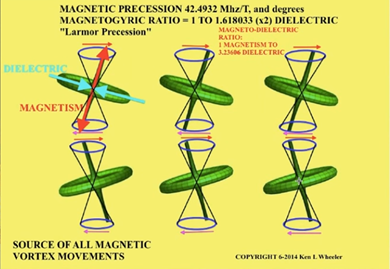Everything gets filtered through the Phi(φ) field with one loci point anchored there. Another gets anchored in the Pi (π) field. We can see in Charles Steinmetz’s notation that even before Tesla he recognized the interaction of Psi and Phi(φ) with the dielectric and magnetic fields.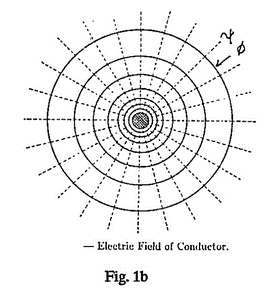The key to our entire existence is dependent on the interaction of the magnetic and dielectric fields, and not just to tan our toast. It permeates the Ether and defines every atom in our known universe. And as we now know, it is based on the 2 Holy Components (27.5 Hz + 15 Hz) = 42.5Hz.

### “Link My Name with the Israelites and I will bless them.”

Connected to the Ark of Salvation are the 15 words and 60 letters of the Blessing of the Cohanim found at Bamidbar 6:2426 with its linkage to the 1820 YHVH (יהוה) in the Torah. The final 8-word 28-letter line, verse 6:27, which occupies the entire 42nd paragraph of Bamidbar, the Book of the 42 Journeys, says, “[The priests] will thus link My Name with the Israelites and I will bless them.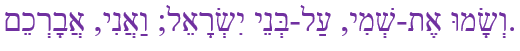The (15 + 8) words and (60 + 28) letters equal (23 + 88) = 111, Alef(אלפ), One (1).

We know where the One (1) cubit skylight is found, the link to His Name.Chag Peseach Sameach!

Ezra